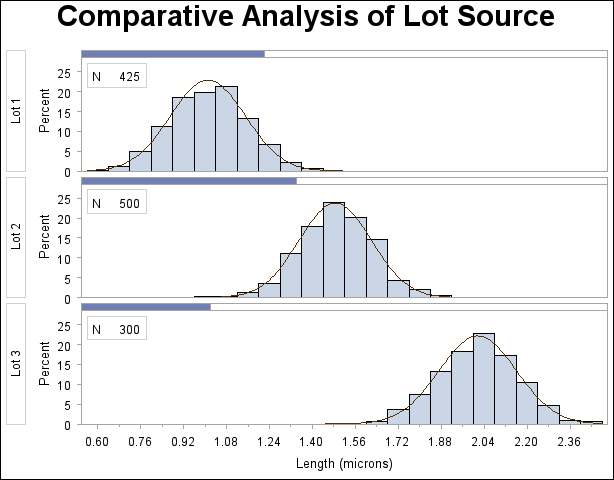The UNIVARIATE Procedure

## Example 4.20 Adding Fitted Normal Curves to a Comparative Histogram

This example is a continuation of Example 4.15, which introduced the data set Channel on . In Output 4.15.3, it appears that the channel lengths in each lot are normally distributed. The following statements use the NORMAL option to fit a normal distribution for each lot:

```title 'Comparative Analysis of Lot Source';
proc univariate data=Channel noprint;
class Lot;
histogram Length / nrows        = 3
intertile    = 1
cprop
normal(noprint);
inset n = "N" / pos = nw;
run;
```

The NOPRINT option in the PROC UNIVARIATE statement suppresses the tables of statistical output produced by default; the NOPRINT option in parentheses after the NORMAL option suppresses the tables of statistical output related to the fit of the normal distribution. The normal parameters are estimated from the data for each lot, and the curves are superimposed on each component histogram. The INTERTILE= option specifies the space between the framed areas, which are referred to as tiles. The CPROP= option requests the shaded bars above each tile, which represent the relative frequencies of observations in each lot. The comparative histogram is displayed in Output 4.20.1.

A sample program for this example, uniex09.sas, is available in the SAS Sample Library for Base SAS software.

Output 4.20.1 Fitting Normal Curves to a Comparative HistogramPrevious Page | Next Page | Top of Page